# Summation Calculator

Enter starting value, ending value, equation, and variable to find the sum of a function using sigma notation calculator.

This will be calculated:

Give Us Feedback

## Summation calculator with Sigma Notation (Σ)

Summation calculator is an online tool that calculates the sum of a given series. It can find the Sigma notation sum of any function. This summation notation calculator also shows the calculation with steps.

## What is summation?

Summation is the process of the addition of a sequence of any type of number. Besides numbers, other types of values such as functions, matrices, and vectors can be summed as well.

Summation is denoted by the Greek letter Sigma notation Σ.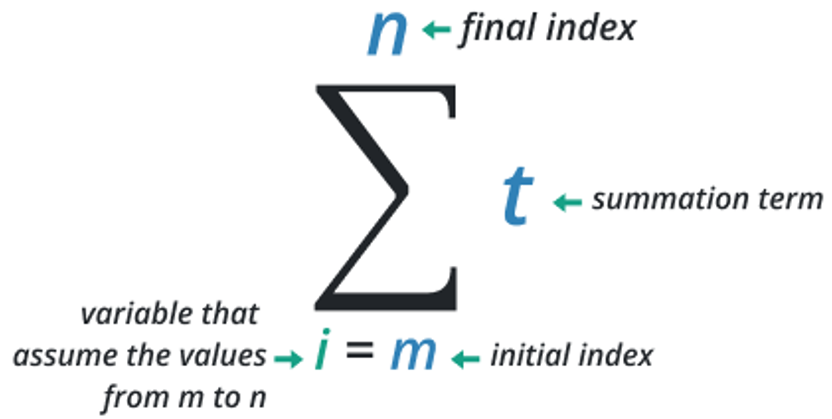## Summation notation formula

The equation to find the sum of the series is given below.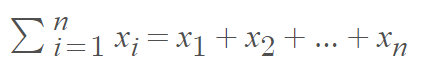Where,

• i is the starting value, and
• n is the upper limit.

## Types of summation

There are two types of summation.

1. Simple summation
2. Sigma Notation
 Type Name Statement Expression Simple Summation It is used to add numbers or quantities arithmetically. It can also be referred to as the algebraic sum of numbers or quantities. 1+2+3+4=10 Sigma Notation The sigma notation is used to evaluate the sum of the function by placing the lower and upper limit values. The lower limit of the summation is said to be the index of the given expression. While the upper limit is said to be the endpoint of the given expression.The problems of the sigma notation can also be solved with the help of our sum of series calculator for well-known functions such as x2, 2x-1, etc. $${\sum _{i=0}^n\left[f\left(x\right)\right]}$$

## How to evaluate summation?

To calculate summation notation, follow the example given below.

Example 1:

Find the sum of the first 10 prime numbers

Solution

Step 1: Write the first 10 prime numbers along with the addition sign between them.

2 + 3 + 5 + 7 + 11 + 13 + 17 + 19 + 23 + 29

Step 2: Now evaluate the sum of the series of prime numbers.

2 + 3 + 5 + 7 + 11 + 13 + 17 + 19 + 23 + 29 = 129

Hence the sum of the first 10 prime numbers is 129.

Example 2:

Evaluate summation for the function (x + 2)2 with an upper limit of 10 and a starting value of 4.

Solution:

Step 1: First of all, let’s identify the values.

x = 4, n = 10

Step 2: Place the given function in the summation equation.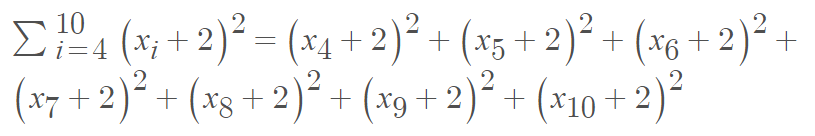Step 3: Substitute the series values in the above equation.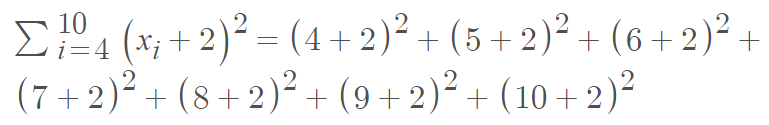Step 4: Simplify the equation.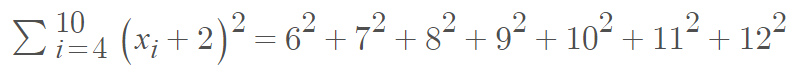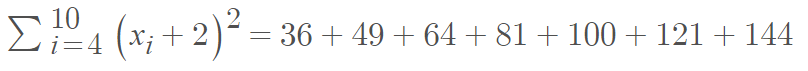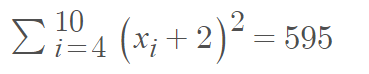## Different formulas for calculating summations

 Summation for Formula Sum of natural numbers mΣx=1 x = [m(m + 1)]/2 Sum of squares of natural numbers mΣx=1 x2 = [m(m + 1)(2m + 1)]/6 Sum of cubes of natural numbers mΣx=1 x3 = [m2(m + 1)2]/4 Sum of 4th power of natural numbers mΣx=1 x4 = [m(m + 1)(2m + 1)(3m2 + 3m – 1)]/30 Sum of 1st m even numbers mΣx=1 2x = m(m + 1) Sum of 1st m odd numbers mΣx=1 (2x + 1) = m2 Sum of an arithmetic sequence mΣx=1 a + (x – 1)d = m[2a + (m – 1)d]/2

### Other Languages